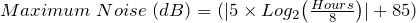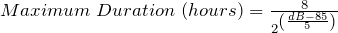HomeCalculatorsMaximum Noise (Decibels) and Time Exposure Calculator

# Maximum Noise (Decibels) and Time Exposure Calculator

Most countries have a stated maximum continuous noise level over a continuous 8 hour period (a working day). This is normally agreed to being a maximum continuous noise level of 85dB for 8 hours.

Some authorities then say for every 3dB increase of continuous noise level, the maximum exposure time is halved.

Other authorities say for every 5dB increase of continuous noise level, the maximum exposure time is halved.

These calculators show results for both the 3dB standard and the 5dB standard.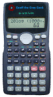Check your local authority to know which standard you should use.

There are two calculators, the first converts the hours of continuous exposure under 8 hours to maximum continuous noise levels (in decibels) over that exposure duration.

The second calculator converts the continuous noise level over 85dB to the maximum continuous exposure time for that noise level.

For those who need to know, the formulas used for the 3dB standard calculations are: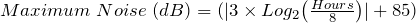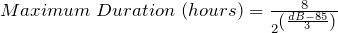and for the 5dB standard calculations: